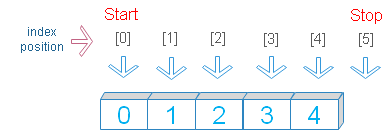# range()

range(start,stop,step) returns a range object with sequence of numbers ( Immutable ).
 start (optional) default = 0 , The start position of the range stop ( required ), range should Stop before this position step ( optional ) default =1 , step to consider for the rangeStart, stop and step values are indices of a range.

Python range() function to generate a range of numbers with start stop and step values

If one value is given then it is considered as stop value as default start is 0 and step value is 1.
If two values are given then it is considered as start and stop values and step value is set to 1
If three values are given then they are considered as start , stop and step values.

## creating a list using range object

We will create one list by using one range object. Here we have used only stop value. x is the range object.
``````x=range(5)      # 5 is stop value
print(list(x))  # [0, 1, 2, 3, 4]``````
With start and stop values
``````x=range(2,5)      # 2 is start ,5 is stop value
print(list(x))    # [2, 3, 4]``````

With start , stop and step values
``````x=range(2,5,2)    # 2 is start ,5 is stop,2 is step value
print(list(x))    # [2, 4]``````

## Using a list to create an range

We will create one list whose elements we will use to create one range. We have to unpack the list to get the elements. What is list unpacking ?
``````my_list=[5,40,10]
for i in range(*my_list):   # unpacking the list inside range
print(i)``````
Output
``````5
15
25
35``````
We can use range to create a list with elements
``````x=range(5,50,10) # range with start=5,stop=50, step=10
my_list=list(x) # list created
print(my_list)``````
Output
``[5, 15, 25, 35, 45]``

## Using negative numbers

``````x=range(5,-10,-3)
print(list(x)) # [5, 2, -1, -4, -7]``````

## range data type

By using type()
``````x=range(5)      # 5 is stop value
print(type(x))  # <class 'range'>``````
range() returns immutable object, so we can't change the elements.

## for loop

We used For loop to display elements.
``````for x in range(5):
print(x)``````
Output
``````0
1
2
3
4``````

Subscribe to our YouTube Channel here

## Subscribe

* indicates required
Subscribe to plus2netplus2net.com